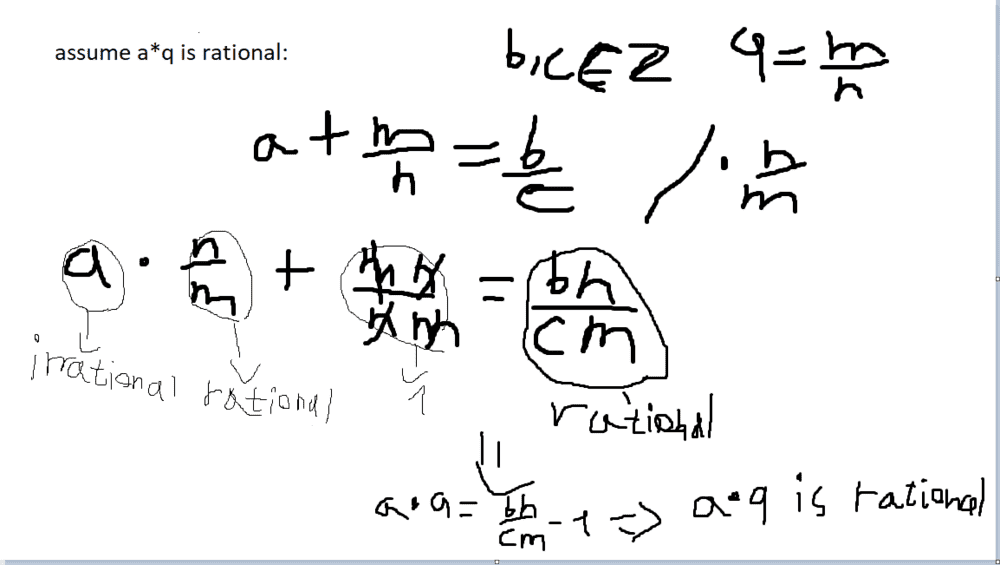# Sum of rational and irrational is irrational

bigchaka
Summary:: i get a proof that sum of rational and irrational is rational
which is wrong(obviously)

let a be irrational and q is rational. prove that a+q is irrational.
i already searched in the web for the correct proof but i can't seem to understand why my proof is false.
my proof:as you can see the proof i get is that the sum is rational.
can someone explain why thi

Mentor
2022 Award
I don't understand why you talk about ##a+q## and write about ##a\cdot q##.
Anyway. What you did is: If ##a+q=q'## then ##a\in \mathbb{Q}##. This is correct. You wrote that ##a## is irrational, but you didn't use it. Quite the opposite is true: you started with an equation where ##a## is already rational.

This is the way to prove it anyway, both additive and multiplicative:

Given ##a\notin \mathbb{Q}## and ##q\in \mathbb{Q}##.
Assume ##a+q \in \mathbb{Q}##. Then ##a+q=q'\in \mathbb{Q}## i.e. ##a=q'-q \in \mathbb{Q}## since ##\mathbb{Q}## is closed under addition. But this contradicts ##a\notin \mathbb{Q}##, hence the assumption ##a+q \in \mathbb{Q}## was wrong, i.e. ##a+q \notin \mathbb{Q}##.

Multiplication goes the same way, except that you have to deal with ##q=0## as special case where the statement is wrong. The proof needs inverse elements and ##0## has no multiplicative inverse.

Last edited:
•bigchaka and etotheipi
bigchaka
I don't understand why you talk about a+q and write about a⋅q.
Anyway. What you did is: If a+q=q′ then a∈Q. This is correct. You wrote that a is irrational, but you didn't use it. Quite the opposite is true: you started with an equation where a is already rational.

This is the way to prove it anyway, both additive and multiplicative:

Given a∉Q and q∈Q.
Assume a+q∈Q. Then a+q=q′∈Q i.e. a=q′−q∈Q since Q is closed under addition. But this contradicts a∉Q, hence the assumption a+q∈Q was wrong, i.e. a+q∉Q.

Multiplication is the same way.
sorry my bad. it was mixed inside my head i did proofs all the day..
you are right.

Mentor
You have shown "if a+q is rational and q is rational then aq is rational". Which is correct, but a+q and q both being rational implies a is rational.

•bigchaka
bigchaka
You have shown "if a+q is rational and q is rational then aq is rational". Which is correct, but a+q and q both being rational implies a is rational.
I got it, thanks!

Staff Emeritus